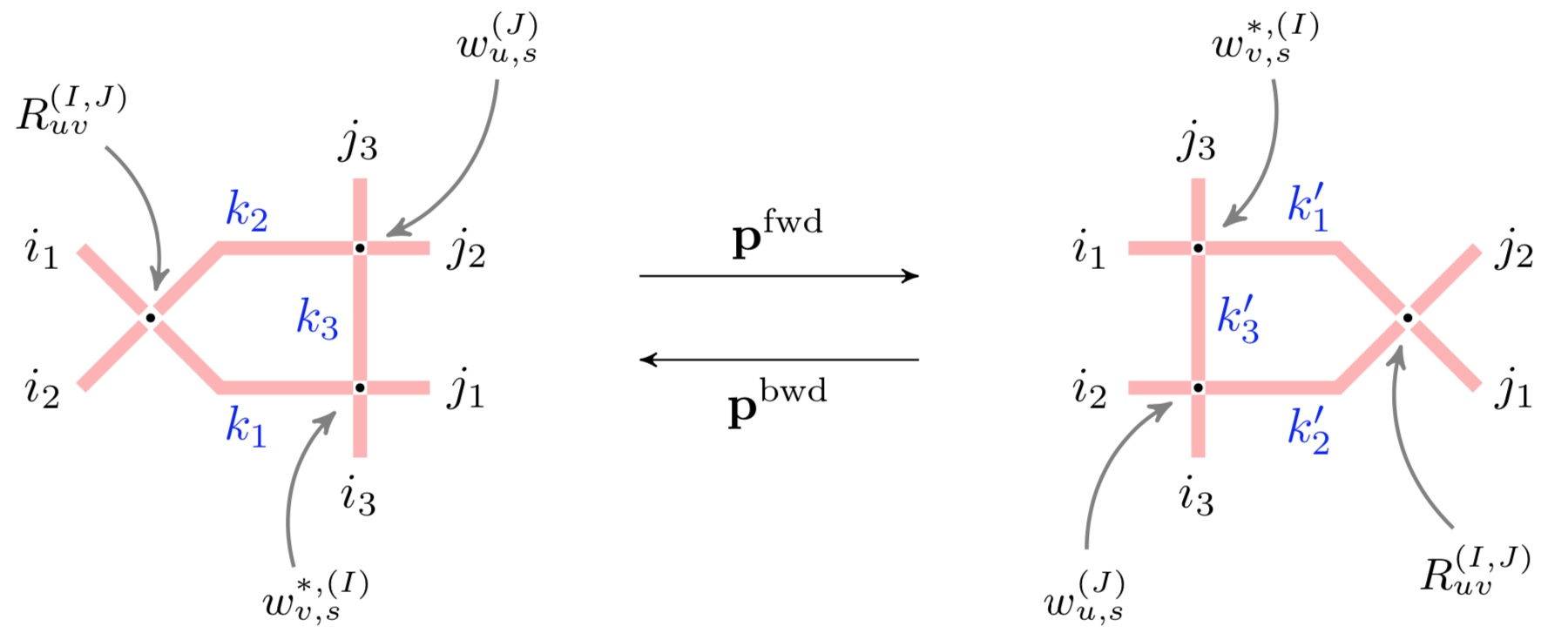# Yang-Baxter random fields and stochastic vertex models

### 2019/05/15

(with Alexey Bufetov, Matteo Mucciconi)
Advances in Mathematics, to appeararXiv:1905.06815 [math.PR]

PDF

Bijectivization refines the Yang-Baxter equation into a pair of local Markov moves which randomly update the configuration of the vertex model. Employing this approach, we introduce new Yang-Baxter random fields of Young diagrams based on spin $q$-Whittaker and spin Hall-Littlewood symmetric functions. We match certain scalar Markovian marginals of these fields with (1) the stochastic six vertex model; (2) the stochastic higher spin six vertex model; and (3) a new vertex model with pushing which generalizes the $q$-Hahn PushTASEP introduced recently by Corwin-Matveev-Petrov (2018). Our matchings include models with two-sided stationary initial data, and we obtain Fredholm determinantal expressions for the $q$-Laplace transforms of the height functions of all these models. Moreover, we also discover difference operators acting diagonally on spin $q$-Whittaker or (stable) spin Hall-Littlewood symmetric functions.Yang-Baxter equation and its bijectivisation